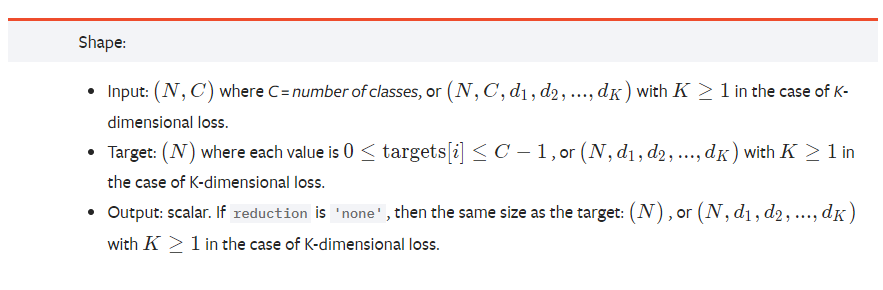## 2020年1月14日星期二

### 1only batches of spatial targets supported (non-empty 3D tensors) but got targets of size: :

I encountered the above problem when using Pytorch's CrossEntropy function.

E.g:
criterion = nn.CrossEntropyLoss()

loss = criterion(logit, target.long())
If logit.shape is torch.Size ([4, 31, 256, 256])  and target.shape is [4, 256, 256, 1], where 4 is the batchSize and 31 is the number of categories.

The error 1only batches of spatial targets supported (non-empty 3D tensors) but got targets of size:: will appear.

### Reason:

Screenshot of Pytorch's CrossEntropy official document is as follows:Obviously, N = 4, C = 31, d1 = d2 = 256, so the 1 in the target is redundant, which is the reason for the error.

### Solution:

loss = criterion(logit, torch.squeeze(target).long())
to change the target to [4, 256, 256].